### Home > CALC > Chapter Ch4 > Lesson 4.5.1 > Problem4-164

4-164.
1. Rewrite the following using a single trigonometric function. You may wish to review your trigonometric identities in Chapter 1. Homework Help ✎

1. 10 sin(3x) cos(3x)

2. sin x cos 3x − sin 3x cos x

3. cos4 x − sin4 x

4. tan x + cot x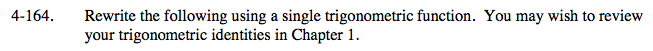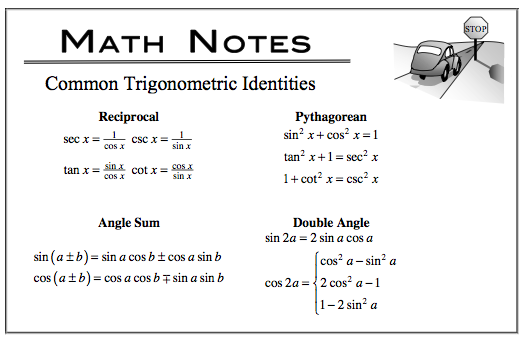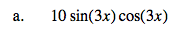Double Angle identity (factor first).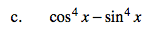Factor first.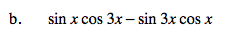Sum and Difference (Angle Sum) identity.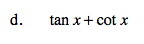Start by rewriting tan(x) and cot(x) as fractions.

You will use more than one identity.

$\frac{\text{sin}x}{\text{cos}x}+\frac{\text{cos}x}{\text{sin}x}=\frac{\text{sin}^{2}x+\text{cos}^{2}x}{(\text{cos}x)(\text{sin}x)}=$

$\frac{1}{\frac{1}{2}\text{sin}(2x)}=2\text{csc}(2x)$